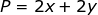## Monday, December 1, 2014

### Square, Rectangle, and Parallelogram Problems, 12

Category: Algebra, Plane Geometry

"Published in Newark, California, USA"

In 1964, Mr. Tambasen bought a rectangular lot in Bacolod City for ₱ 18,000 and spent ₱ 1,000 for building a wall around it. If the cost per square meter of the lot is ₱ 30 and the cost per linear meter of the wall is ₱ 10, find the dimensions of the lot.

Solution:

To illustrate the problem, it is better to draw the figure as followsPhoto by Math Principles in Everyday Life

Let x = be the length of a rectangular lot
y = be the width of a rectangular lot

The area of a rectangular lot is.

The perimeter of a rectangular lot is.

The first working equation which is the cost of a rectangular lot is

The second working equation which is the total cost of building a wall around the rectangular lot is

Substitute the value of y to the first working equation, we have

By using the completing the square method, the value of x which is the length of a rectangular lot is

If you choose the positive sign, the value of x is

and the value of y which is the width of a rectangular lot is

If you choose the negative sign, the value of x is

and the value of y which is the width of a rectangular lot is

Therefore, the dimensions of a rectangular lot are 30 m by 20 m